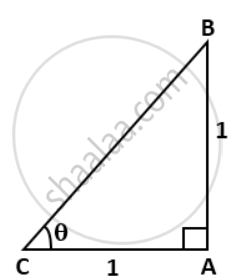# In tan θ = 1, find the value of 5cot2θ + sin2θ - 1. - Mathematics

Sum

In tan θ = 1, find the value of 5cot2θ + sin2θ - 1.

#### SolutionConsider ΔABC, where ∠A = 90°

tan θ = "Perpendicular"/"Basse" = "AB"/"AC" = 1 = (1)/(1)

By Pythagoras theorem,
BC2
= AB2 + AC2
= 12 + 12
= 2
⇒ BC = sqrt(2)
Now,

cot θ = (1)/"tan θ" = 1

sin θ = "AB"/"BC" = (1)/sqrt(2)

∴ 5cot2θ + sin2θ - 1

= 5 xx (1)^2 + (1/sqrt(2))^2 - 1

= 5 + (1)/(2) - 1

= 4 + (1)/(2)

= (9)/(2).

Concept: Reciprocal Relations
Is there an error in this question or solution?

#### APPEARS IN

Frank Class 9 Maths ICSE
Chapter 26 Trigonometrical Ratios
Exercise 26.1 | Q 18